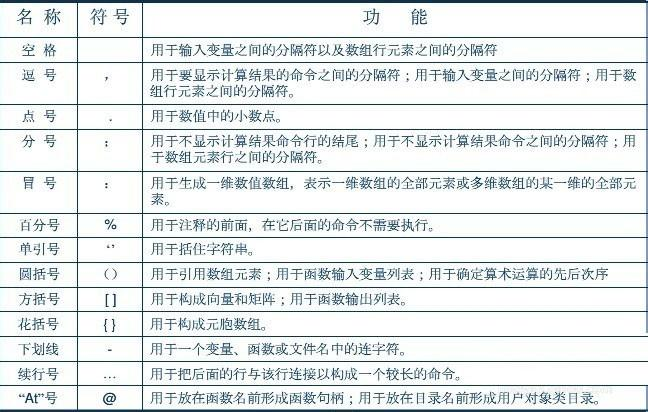• Matlab中,冒号“:”和分号“;”等常用标点符号分别有什么功能?...matlab中分号、冒号、逗号等常用标点符号的作用如图，供学习参考！其中冒号的常用法总结 ：1)a:b 表示[a,a+1,……,b]>> A=1:6A =1 2 ...
在Matlab中,冒号“:”和分号“;”等常用标点符号分别有什么功能?应该怎么使用？接下来小编就给大家分享matlab中分号、冒号、逗号等常用标点符号的功能和用法总结，教你正确使用Matlab中的标点符号。matlab中分号、冒号、逗号等常用标点符号的作用如图，供学习参考！其中冒号的常用法总结 ：1)a:b   表示[a,a+1,……,b]>> A=1:6A =1     2     3     4    5    62)当然如果b和a不是整数的话，则向量的最后一位数是n+a，且n=fix(b-a)>> A=1.2:5.2A =1.2000    2.2000    3.2000    4.2000    5.20003)上面的前提是ab则会出现空值>> A=6:1A =Empty matrix: 1-by-0此时 A是空矩阵，从workspace也可以看到A4)a:c:b表示[a,a+c,……,a+n*c],其中n=fix((b-a)/c)>> A=1:3:11A =1     4     7    105)A(:)表示以一列的方式显示A中所有元素>> A=[1 2 3;4 5 6]A =1     2     34     5     6>> A(:)ans =1425366)b=A(i, :)表示把矩阵A的第i行存入b>> b=A(1,:)b =1     2     37)b=A( :,j)表示把矩阵A的第j列存入b>> b=A(:,1)b =148)b=A(J :K)表示把矩阵A中[A(J),A(J+1),...,A(K)]这些元素存入b中>> b=A(3:5)b =2     5     39)b=A(:,c:d)表示把矩阵A的第c到第d列存入b中，当然c，d大于A的列数则出错(b=A(c:d,:)表示取行)>> A=[1 2 3;4 5 6;7 8 9]A =1     2     34     5     67     8     9>> b=A(:,2:3)b =2     35     68     9教程结束，以上就是关于matlab中分号、冒号、逗号等常用标点符号的功能和用法总结，希望大家看完有所收获!更多matlab相关教程，请继续关注我们网站！
展开全文• matlab中分号、冒号、逗号等常用标点符号的作用，供学习参考 其中冒号的常用法总结 ： 1）a:b 表示[a,a+1,……,b] >> A=1:6 A =  1 2 3 4 5 6 2）当然如果b和a不是整数的话，则向量的最后一...
matlab中分号、冒号、逗号等常用标点符号的作用，供学习参考

其中冒号的常用法总结 ：

1）a:b   表示[a,a+1,……,b]

>> A=1:6

A =

1     2     3     4    5    6

2）当然如果b和a不是整数的话，则向量的最后一位数是n+a，且n=fix（b-a）

>> A=1.2:5.2

A =

1.2000    2.2000    3.2000    4.2000    5.2000

3）上面的前提是a<b，如果a>b则会出现空值

>> A=6:1

A =

Empty matrix: 1-by-0

此时 A是空矩阵，从workspace也可以看到A

4）a:c:b表示[a,a+c,……,a+n*c],其中n=fix((b-a)/c)

>> A=1:3:11

A =

1     4     7    10

5)A(:)表示以一列的方式显示A中所有元素

>> A=[1 2 3;4 5 6]

A =

1     2     3

4     5     6

>> A(:)

ans =

1

4

2

5

3

6

6）b=A(i, :)表示把矩阵A的第i行存入b

>> b=A(1,:)

b =

1     2     3

7）b=A( :,j)表示把矩阵A的第j列存入b

>> b=A(:,1)

b =

1

4

8）b=A(J :K)表示把矩阵A中[A(J),A(J+1),...,A(K)]这些元素存入b中

>> b=A(3:5)

b =

2     5     3

9）b=A（:,c:d）表示把矩阵A的第c到第d列存入b中，当然c，d大于A的列数则出错（b=A（c:d,:）表示取行）

>> A=[1 2 3;4 5 6;7 8 9]

A =

1     2     3

4     5     6

7     8     9

>> b=A(:,2:3)

b =

2     3

5     6

8     9


展开全文冒号
• matlab中分号、冒号、逗号等常用标点符号的作用如图，供学习参考 其中冒号的常用法总结 ： 1）a:b 表示[a,a+1,……,b] >> A=1:6 A =  1 2 3 4 5 6 2）当然如果b和a不是整数的话，则向量...

matlab中分号、冒号、逗号等常用标点符号的作用如图，供学习参考其中冒号的常用法总结 ：

1）a:b   表示[a,a+1,……,b]

>> A=1:6

A =

1     2     3     4    5    6

2）当然如果b和a不是整数的话，则向量的最后一位数是n+a，且n=fix（b-a）

>> A=1.2:5.2

A =

1.2000    2.2000    3.2000    4.2000    5.2000

3）上面的前提是a<b，如果a>b则会出现空值

>> A=6:1

A =

Empty matrix: 1-by-0

此时 A是空矩阵，从workspace也可以看到A

4）a:c:b表示[a,a+c,……,a+n*c],其中n=fix((b-a)/c)

>> A=1:3:11

A =

1     4     7    10

5)A(:)表示以一列的方式显示A中所有元素

>> A=[1 2 3;4 5 6]

A =

1     2     3

4     5     6

>> A(:)

ans =

1

4

2

5

3

6

6）b=A(i, :)表示把矩阵A的第i行存入b

>> b=A(1,:)

b =

1     2     3

7）b=A( :,j)表示把矩阵A的第j列存入b

>> b=A(:,1)

b =

1

4

8）b=A(J :K)表示把矩阵A中[A(J),A(J+1),...,A(K)]这些元素存入b中

>> b=A(3:5)

b =

2     5     3

9）b=A（:,c:d）表示把矩阵A的第c到第d列存入b中，当然c，d大于A的列数则出错（b=A（c:d,:）表示取行）

>> A=[1 2 3;4 5 6;7 8 9]

A =

1     2     3

4     5     6

7     8     9

>> b=A(:,2:3)

b =

2     3

5     6

8     9

展开全文• ## Matlab分号的使用

千次阅读 2018-09-24 19:56:27
运行matlab程序的时候，程序变量的值会自动储存在 workspace。 程序命令后面输入与不输入“;”的区别是，当输入半角的分号时，命令的变量会自动列表储存在 workspace，变量的值不会在window command显示...
后面的加分号时，运行结果在命令窗口不显示。 不加分号时，当点击回车时，运行结果就会立即显示出来！ 运行matlab程序的时候，程序中变量的值会自动储存在 workspace中。

程序命令后面输入与不输入“;”的区别是，当输入半角的分号时，命令中的变量会自动列表储存在 workspace中，变量的值不会在window command中显示出来；当不输入半角的分号时，变量不仅也会自动储存在workspace中，还会在window command窗口中显示出变量的值。


展开全文• 分号MATLAB和python的区别 分号MATLAB中表示语句结果不显示，在python中用于分隔语句，如果要在一行书写多条句，就必须使用分号分隔每个语句，否则Python无法识别语句之间的间隔。
• 运行matlab程序的时候，程序变量的值会自动储存在 workspace。程序命令后面输入与不输入“;”的区别是，当输入半角的分号时，命令的变量会自动列表储存在 workspace，变量的值不会在window command显示...
• 刚接触 matlab的新手一定会有这样的疑问，为什么有些语句是以...在matlab中，如果语句的结尾是逗号，则会把赋值语句输出到命令窗口；分号不会。 比如运行 A=100, B=50; 则命令窗口上会输出 A=100 B不会输出。 OK了吗~
• 今天小鱼分享的比较简单，但是自己老搞混，所以记录下来，方便...看到这里就应该可以知道，matlab中行和列的分开标志是找逗号，有逗号就是行列分开，但如果没有逗号是什么意思呐，看下面 已行的形式表示并取第i行到
• 使用括号[]表示数组时，分号;用来隔开行。用法如图。 使用括号[]还可以拼接数组。如下，我们有两个数组A和B. [A,B]相当于水平拼接A和B，即horzcat(A,B) [A;B]相当于垂直拼接A和B，即vertcat(A,B) 具体见以下...
• $(".cart").droppable({ drop: function () {$(this)//这里为什么不能加分号？？？？ .addClass("focus")//这里为什么不能加分号？？？？ .find("#tip").html(""); } }) }); 搞不清楚什么时候该加什么时候不该...
• 在运行matlab程序代码时，程序的变量的值都会自动存储在workspace。 而分号是否加的区别在于：代码后加分号，运行结果不会显示在命令窗口； 代码后不加分号，当点击回车时，运行结果会立即在命令窗口（window ...
• 在代码行的后加分号，运行结果不会显示在命令行窗口； 不加分号，变量的值会随着运行显示在...如果不想命令行窗口显示太多东西，可以在代码编写的过程加上分号，需要知道变量的值的时候在工作区进行查看即可。 ...
• 初学者刚学习MATLAB的时候，首先有必要了解MATLAB的基本语法以及MATLAB中的常量和变量。在学习MATLAB中的常量和变量之前首先我们有必要了解一下MATLAB基本的语法。如下图所示，进入命令窗口，每行语句前都有一个符号...
• 工具/材料Matlab首先在Matlab中最常用的声明数组的方式就是使用括号和分号了，如下图所示然后我们也可以把括号去掉，改成手动换行，如下图所示，括号在声明数组的时候就是起到了换行的作用接下来我们还可以用...
• MATLAB中创建一个字符串非常简单。 事实上，在前面的示例我们已经使用了很多次。 例如，在命令提示符下键入以下内容：my_string = 'Yiibai Yiibai'MATLAB执行上述语句并返回以下结果 -Trial>> my_string =...
• MATLAB - Strings在MATLAB中创建字符串非常简单。 事实上，我们已多次使用它。 例如，在命令提示符下键入以下内容 -my_string = 'IOWIKI'MATLAB将执行上述语句并返回以下结果 -my_string = IOWIKIMATLAB将所有变量视...
• 3.分号 （1）用于指令后，不显示计算结果 A=[1 2 3];B=[0 0 1] B = 0 0 1 （2)用于矩阵，作为行与行之间的分隔符 A=[6 5 4;7 8 9] A = 6 5 4 7 8 9 4.百分号用于注释 5.大括号  ...
• 此函数根据配置文件数据查找产生非抑制输出（不以分号终止）的代码行。 只考虑实际调用的函数。 使用： 简介; YourFunct(a,b,c); 配置文件关闭； p=profile('信息'); findNonSuppressedOutput( p, '/code/...
• MATLAB中定义赋值一个二维数组答： 分号；;因特网能提供的最基本服务答：E-mail, WWW, FTP劳动合同，用人单位给予劳动者低于当地工资最低水平的违反了()合法原则答：内容合法中国大学MOOC: ZXMP S320设备的PDH接口...
• a=[1:5]' %冒号 b=cumsum（[1:a]）%冒号 产生一个值 b=cumsum（[1;a]） %分号 产生6×1个值 注意区分matalb的冒号和分号，看起来很相似~
• 不能在统一目录下共存同名的.m文件和.mat文件，且在该.m文件同时加载了该.mat文件，即这样，虽然加了分号，但是或许时matlab的运行机制，会导致重复载入mat文件且是无分号的效果。 解决方案就是，将两文件bug
• 下面来介绍一下MATLAB中一些比较常见的矩阵运算，这也是我们进行算法调试的基础，具体如下所示： 1、显示矩阵A：在主界面的命令行窗口输入下列代码： A = [1 3 5;1 0 1;5 0 9] 方括号内是矩阵A的元素，每行...矩阵运算
• 具体用法如下： 1、a:b 表示[a,a+1,……,b] >> A=1:6 A = 1 2 3 4 5 6 2、当然如果b和a...7 8 9] A = 1 2 3 4 5 6 7 8 9 >> b=A(:,2:3) b = 2 3 5 6 8 9 扩展资料 matlab中分号、冒号、逗号等常用标点符号的作用如图
• ## matlab中括号

千次阅读 2018-01-05 17:23:44
括号用来构建向量(Vectors)或者是矩阵(Matrices)。如[6.9 9.64 sqrt(-1)] 就是一个有三个元素的向量。...Matlab 方括号“[ ]”的作用 1定义矩阵（向量，数组）。 例如 a=[1 2 3;4 5 6] 2.定义函数的返回
• 在 'linevals' 返回一个结构，其中包含行号和可能缺少终端分号的候选行的冒犯字符串。 以通常不会在命令行产生输出的关键字（例如，'if'、'return'、'continue' 等）开头的行将被跳过。 TARGETFILES：输入可以是...
• ## matlab中的括号

千次阅读 2015-10-23 14:25:28
Matlab中经常会用到括号去引用某Array或者是cell的内容，但三者有什么具体区别呢？ [ ] 括号用来构建向量(Vectors)或者是矩阵(Matrices)。如[6.9 9.64 sqrt(-1)] 就是一个有三个元素的向量。 [11 12 13; 21 22...括号
• 经过前几篇的学习，我想对于Matlab的两种M文件都已经清楚各自的功能并对函数的相关知识有了一定的了解，今天这篇将对Matlab的程序控制结构进行学习，首先要知道在Matlab中，具有四类程序控制结构方式，依次是：条件...
• MATLAB02:结构化编程和函数定义结构化编程流程控制语句和逻辑运算符流程控制语句示例使用循环语句应尽量预先分配内存空间编写脚本时应注意的问题在脚本开头应添加语句清空工作区在运算和赋值语句后应添加分号`;......

# matlab中分号的作用matlab 订阅